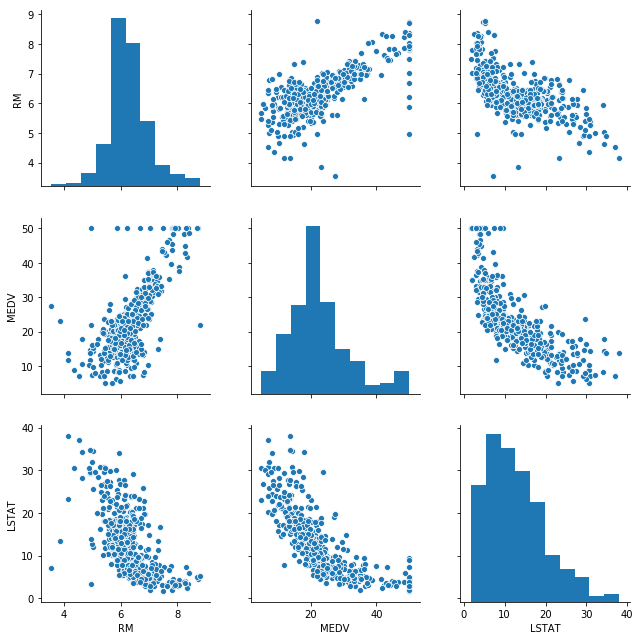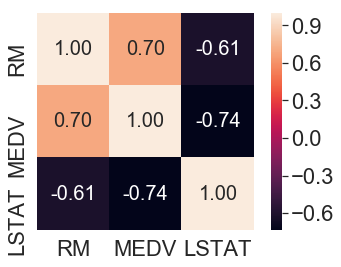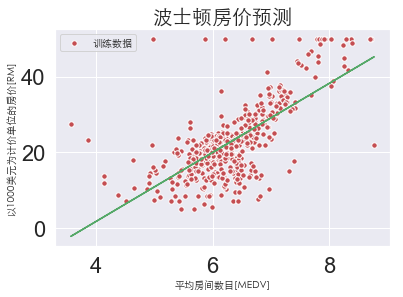# 一、导入模块

import pandas as pd
import numpy as np
import matplotlib.pyplot as plt
import seaborn as sns
from matplotlib.font_manager import FontProperties
from sklearn.linear_model import LinearRegression
%matplotlib inline
font = FontProperties(fname='/Library/Fonts/Heiti.ttc')


# 二、获取数据

housing-data.txt数据下载地址https://pan.baidu.com/s/1SvG0hFxupYA3KQO4fLsP5A

## 2.1 打印数据

df = pd.read_csv('housing-data.txt', sep='\s+', header=0)

CRIM ZN INDUS CHAS NOX RM AGE DIS RAD TAX PTRATIO B LSTAT MEDV
0.00632 18.0 2.31 0 0.538 6.575 65.2 4.0900 1 296.0 15.3 396.90 4.98 24.0
0.02731 0.0 7.07 0 0.469 6.421 78.9 4.9671 2 242.0 17.8 396.90 9.14 21.6
0.02729 0.0 7.07 0 0.469 7.185 61.1 4.9671 2 242.0 17.8 392.83 4.03 34.7
0.03237 0.0 2.18 0 0.458 6.998 45.8 6.0622 3 222.0 18.7 394.63 2.94 33.4
0.06905 0.0 2.18 0 0.458 7.147 54.2 6.0622 3 222.0 18.7 396.90 5.33 36.2

# 三、特征选择

## 3.1 散点图矩阵

# 选择三列特征
cols = ['RM', 'MEDV', 'LSTAT']
# 构造三列特征之间的联系即构造散点图矩阵
sns.pairplot(df[cols], height=3)
plt.tight_layout()
plt.show()## 3.2 关联矩阵

# 求解上述三列特征的相关系数
'''

'''
cm = np.corrcoef(df[cols].values.T)
# 控制颜色刻度即颜色深浅
sns.set(font_scale=2)
# 构造关联矩阵
hm = sns.heatmap(cm, cbar=True, annot=True, square=True, fmt='.2f', annot_kws={
'size': 20}, yticklabels=cols, xticklabels=cols)
plt.show()# 四、训练模型

X = df[['RM']].values
y = df['MEDV'].values

lr = LinearRegression()
lr.fit(X, y)

LinearRegression(copy_X=True, fit_intercept=True, n_jobs=None,
normalize=False)


# 五、可视化

plt.scatter(X, y, c='r', s=30, edgecolor='white',label='训练数据')
plt.plot(X, lr.predict(X), c='g')
plt.xlabel('平均房间数目[MEDV]', fontproperties=font)
plt.ylabel('以1000美元为计价单位的房价[RM]', fontproperties=font)
plt.title('波士顿房价预测', fontproperties=font, fontsize=20)
plt.legend(prop=font)
plt.show()
print('普通线性回归斜率:{}'.format(lr.coef_))普通线性回归斜率:9.10210898118031


posted @ 2019-10-16 17:07  二十三岁的有德  阅读(5540)  评论(0编辑  收藏  举报